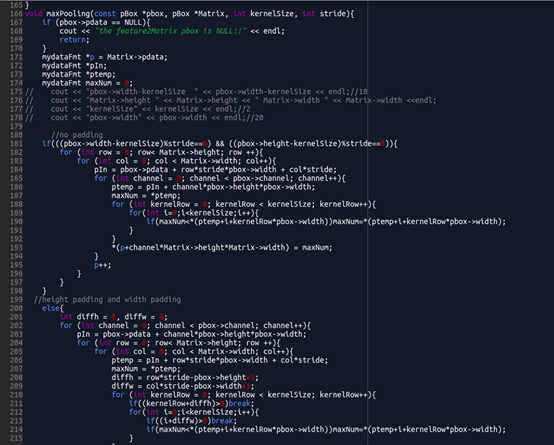# 摘要

MTCNN是一个优秀的人脸检测模型，在网上有各种框架下的版本，在项目中需要使用MTCNN的C代码版本，该版本的作者并没有提供生成代码运行的txt权重参数文件的程序，同时使用txt文件来存参数，文件的体积比较大，在项目中我们需要使用tensorflow训练出来的权重参数给模型运行，因此就涉及到如何把tensorflow训练出来的权重文件转化为bin文件，用于替代程序中的txt文件

# 如何生成bin文件

PnetOutFile = "Pnet.bin"
PnetBinFile = open(PnetOutFile,'wb')
PnetBinFile.write(struct.pack('f', Pconv1_w[y,x,i,j]))
PnetBinFile.close()


# 权重文件写入顺序

## 1、conv层权重参数

Pconv1_w = sess.run(pnet_var)
ky, kx, num_in_map, num_out_kerns = Pconv1_w.shape
print(0,Pconv1_w.shape)
for j in range(num_out_kerns):
for i in range(num_in_map):
for y in range(ky):
for x in range(kx):
PnetBinFile.write(struct.pack('f', Pconv1_w[y,x,i,j]))


## 2、conv层偏置、prelu层的写入

#Rconv1_b
Rconv1_b = sess.run(rnet_var)
print(1,Rconv1_b.shape)
for item in range(0,len(Rconv1_b[:])):
RnetBinFile.write(struct.pack('f',Rconv1_b[item]))
#RPReLU1
RPReLU1 = sess.run(rnet_var)
print(2,RPReLU1.shape)
for item in range(0,len(RPReLU1[:])):
RnetBinFile.write(struct.pack('f',RPReLU1[item]))


## 3、fc层

#Rconv4_w  (576,128)
Rconv4_w = sess.run(rnet_var)
print(9,Rconv4_w.shape)
num_in_map, num_out_kerns = Rconv4_w.shape
RfcTemp = []
RfcTemp2 = []
for j in range(num_out_kerns):#128
for i in range(num_in_map):#576
RfcTemp.append(Rconv4_w[i,j])
for n in range(64):
for k in range(9):
RfcTemp2.append(RfcTemp[n + k*64])
for l in range(576):
RnetBinFile.write(struct.pack('f', RfcTemp2[l]))
RfcTemp = []
RfcTemp2 = []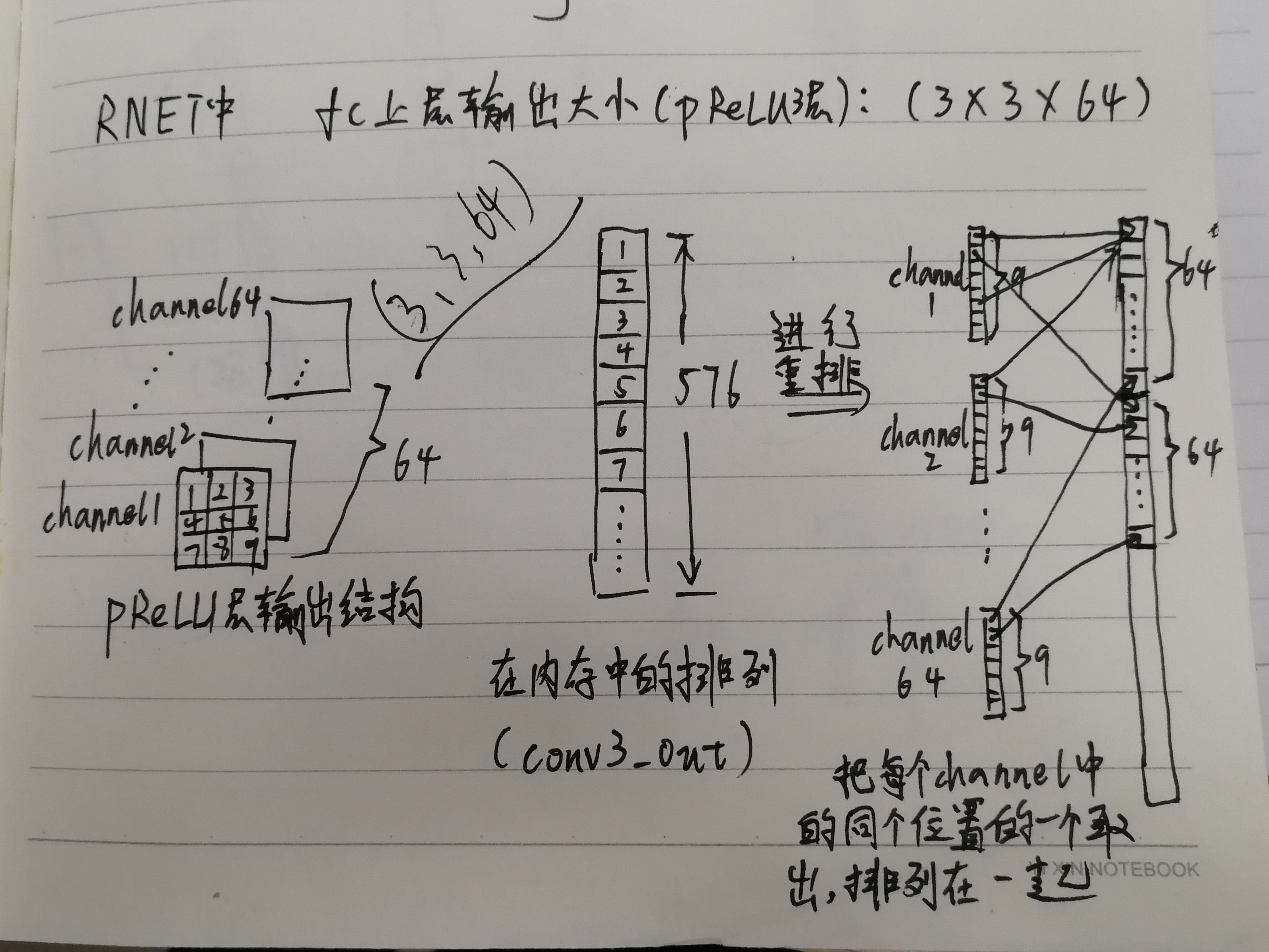# 调试方法介绍

    feature2Matrix(this->rgb, this->conv1_matrix, this->conv1_wb);
convolution(this->conv1_wb, this->rgb, this->conv1_out, this->conv1_matrix);
prelu(this->conv1_out, this->conv1_wb->pbias, this->prelu_gmma1->pdata);
pBoxShow(this->conv1_out);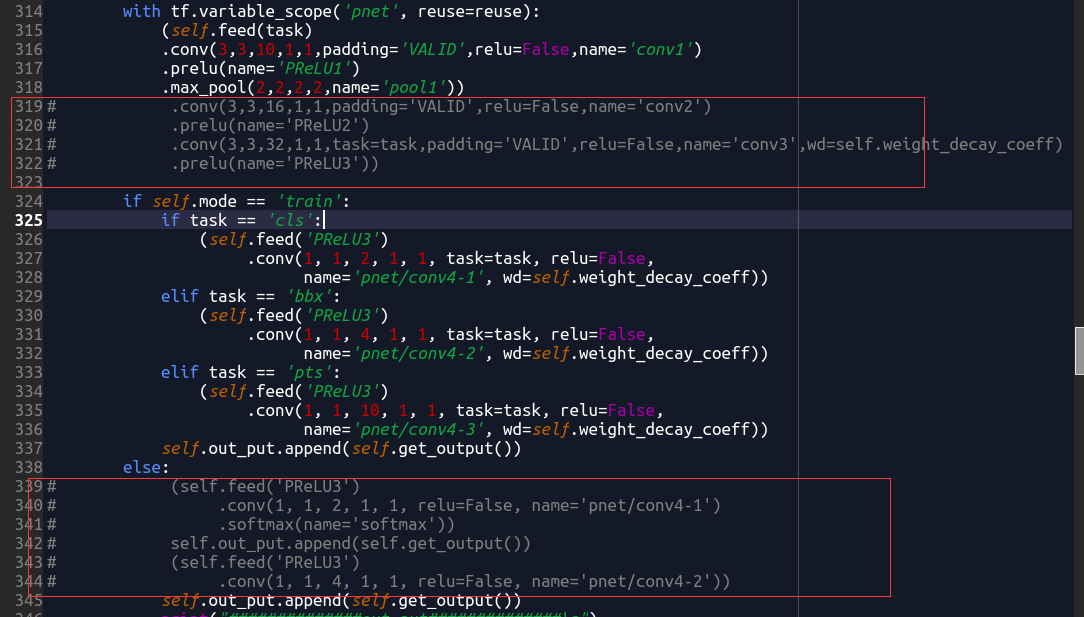out = pnet(img)
im_data = np.array(out)
for c in range(3):
print("channel:",c)
tempImg = []
for y in range(17):
for x in range(21):
tempImg.append(im_data[y,x,c])
print(tempImg)
tempImg = []
print("\n")


# C代码修改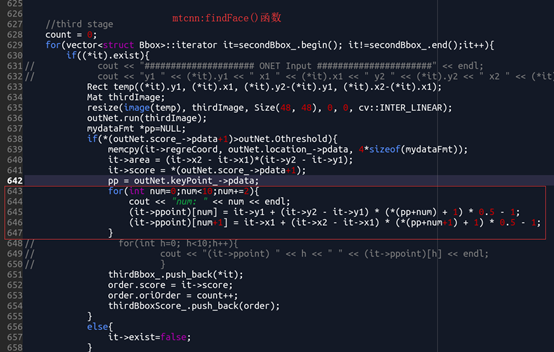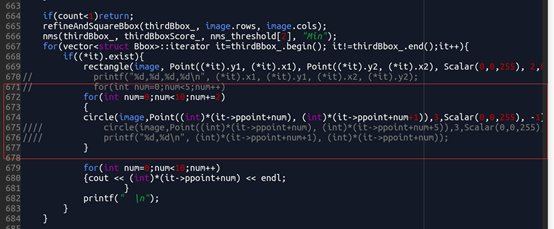# 其他问题

C代码中也有一些缺陷：
network.cpp代码中的maxpooling（）函数有缺陷，在pooling过程中如果遇见需要补零的feature map，只对x方向补零，却没有y方向补零，导致最后一行的maxpooling输出有差错